# Kinematics

## Kinematics

The following text is used only for teaching, research, scholarship, educational use and informative purpose following the fair use principles.

We thank the authors of the texts and the source web site that give us the opportunity to share their knowledge

# Kinematics

Kinematics is the study of motion.  We will use the concepts of displacement, velocity and acceleration to describe this motion mathematically.

The one note I’d like to make clear is that you need to specify your origin clearly.  For consistency, I will always choose the origin to be the initial position of the object.  To determine whether or not something is positive or negative, just draw a coordinate system and you’ll see.  If an object moves to the right or up from the origin, you have positive motion. If an object moves to the left or down from the origin, it is negative motion, just like a number line.

To break this down: a positive displacement means an object’s final position is to the right or up from its initial position.  A negative displacement is when an objects final position is to the left or down from its initial position.

A positive velocity is when the object is moving up or to the right (it doesn’t matter where it is, just where its going.)  A negative velocity is when the object is moving down or to the left.  So, even if you have an object with a negative displacement, it could have a positive or negative velocity.

A negative acceleration, for instance, could refer to an object slowing down going to the right or speeding up towards the left.

Displacement is an object’s change in position.  An object’s displacement is the vector that points from an object’s initial position to its final position, regardless of the path actually taken.

Speed versus Velocity – velocity is the rate of change of position.  Note that speed is a scalar quantity and velocity is a vector quantity (magnitude and direction).

To find average speed or average velocity:

average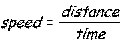average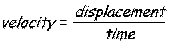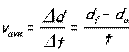While average speed and average velocity will usually be equivalent in magnitude, they can differ.  Remember that for velocity, you are only using change in displacement, which means final position- initial position, regardless of the path taken; whereas for speed, you will be using the total distance traveled.

Instantaneous velocity is the velocity at one particular instant in time, not an average. This is what your car speedometer reads.

Acceleration is the rate of change of velocity (change in speed or direction). For now, we will only be considering constant acceleration.  These equations do not apply to a changing acceleration (like one that changes with time or position).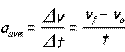This equation can be rewritten as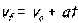With some algebraic manipulation, you can derive the following equations: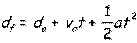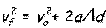Depending on the situation, you will need to use different equations. The way to determine which equation to use is by looking at your knowns and unknowns.  Many problems can be solved using a variety of different combinations, so there isn’t always one right method for each problem.

Kinematics with Graphs

You’ll be doing a bunch of these in calculus and we’ll come back to this later, but there are some basic rules that you need to know about graphs for constant acceleration.

In a position versus time graph:
-the slope of the graph will yield average velocity (because slope is the change in position/the change in time or the rate of change of position)
- to get the instantaneous velocity, you need to find the slope between very short time periods

In a velocity versus time graph:
-the slope is acceleration (because acceleration is the rate of change of velocity)
-the area under the curve (+ if above the horizontal axis and – if below) will give you the displacement (because velocity x time yields displacement)

In an acceleration versus time graph:
-the area under the curve will give you the increase in velocity from the initial velocity (because final velocity = initial velocity + acceleration x time).

Free Fall is the effect of objects falling only under the influence of gravity. This is a prime example of constant acceleration.  In free fall, objects accelerate towards the earth at 9.8 m/s2.  What that means is that an object in free fall will speed up by 9.8 m/s every second.  You can solve free fall problems as basic kinematics problems using the equations above, substituting in a = g= -9.8m/s2.

If you throw an object up, the initial velocity is positive, but once it starts coming down, the velocity becomes negative, even though the displacement is still positive. The displacement it goes above the initial position is positive, and any displacement it goes below the initial position is negative.  During the whole route, the acceleration is negative (it’s always down).

Two Dimensional Motion

2-d motion follows the same rules as 1-d motion, but you need to remember to keep your vertical and horizontal components separated.  We call this the independence of vertical and horizontal motion, which means the motion in the horizontal direction doesn’t affect the motion in the vertical direction and vice versa.  Let’s first look at an example of a function using unit vectors.

(i’s, j’s and k’s should have carrot tops because they are unit vectors)

at t=0
Position do= 5m i + 2 m j
vo = 3m/s i + 4 m/s j
ao = 2 m/s2 i + 1 m/s2 j

Find the velocity and position at t=3 seconds.
v(t)    = vo + at
v(3)   = 3m/s i + 4 m/s j + (2 m/s2 i + 1 m/s2 j) (3s)
=3m/s i + 4 m/s j + 6 m/s i + 3 m/s j)
= 9m/s i + 7m/s j

d(t)    = do + vot + ½ at2
d(3)   = 5m i + 2 m j + (3m/s i + 4 m/s j) (3s) + ½ (2 m/s2 i + 1 m/s2 j) (3s)2
= 5m i + 2 m j + 9m i + 12 m j + 9 m i + 4.5 m j
= 23m i + 18.5 m jAnd if you needed to find the magnitude and direction of that vector, you can just draw it out, do the Pythagorean Theorem and your trig functions, and you’ll get that it is 29.52 m at an angle of 520 from the horizontal.

Projectile Motion utilizes these two dimensional rules with the distinction that the vertical dimension is determined by the acceleration due to gravity.

The first thing to do is to split up the initial velocity into its horizontal and vertical components.Voy = Vo sin q
Vox = Vo cos q

From here, you can split up the motion into two separate pieces.

Horizontal (assuming no air resistance)                 Vertical
xf = xo + voxt                                                          yf = yo + voyt + ½ gt2
vox = vfx                                                                                                             vfy =  voy + gt
ax= 0                                                                    vfy2 =  voy2 + 2gDy
ay= -9.8 m/s2

From this point, you need to determine what information you are given to know which equations to use.  Just remember that the horizontal and vertical components can be treated separately.  The only thing that they share in common is the time.

Source : http://www.groovytoo.com/robinson/AP/kinematics%20notes.doc

Author : Ms. Robinson

If you are the author of the text above and you not agree to share your knowledge for teaching, research, scholarship (for fair use as indicated in the United States copyrigh low) please send us an e-mail and we will remove your text quickly.

### Kinematics

#### Kinematics

Main page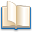Alimentation - nutritionAnimalsArchitectureArtAstrology, esoteric, parapsychology and fantasyAstronomyBiology and geneticsBotany and agricultureChemistryCourses, training, guides, handbooks and tipsCulture General and actualityDermatology, health and wellnessDictionaries and glossariesEconomics and financeEducation and pediatricsEngineering and technologyEnglish grammar and anthologyFashion and show businessFitnessFood, recipes and drinkGames, play spaceGeography, geology and environmentHistoryInformatics and computer worldJobs worldLanguagesLeisure and DIY do it yourselfLessons for studentsLiterature, biographies and journalismMathematics, geometry and statisticalMedicineMusicNotes and concept mapsPhilosophyPhotography and images - picturesPhysicsPhysiologyPsychology and psychoanalysisQuiz questions and answersReligionsRights law and political scienceScienceSociology and cultural anthropologySportSummaries and reviewsTelevision, film - movies and comicsTheater and communicationsTheses, themes and dissertationsTravel and tourist guidesUseful documents and tablesUtility, calculators and convertersWeather and meteorology

#### Kinematics

This is the right place where find the answers to your questions like :

Who ? What ? When ? Where ? Why ? Which ? How ? What does Kinematics mean ? Which is the meaning of Kinematics?

##### Kinematics

Alanpedia.com from 1998 year by year new sites and innovations